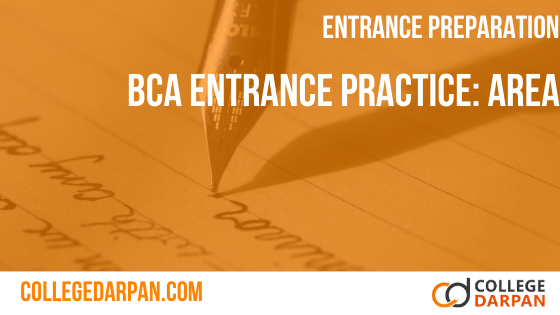# BCA Entrance Practice Quiz: Area

1. A rectangular carpet has an area of 120 square meters and a perimeter of 46m. The length of its diagonals is_________?
2. The perimeter of a floor of a room is 18m.What is the area of four walls of the room if it; a height is 3m?
3. A rectangular measure 8cm on length and it’s diagonals measures 10cm what is the perimeter of the rectangular?
4. A diagonal of rectangle is √41cm and area is 20 sq. cm. The perimeter of the rectangle must be.
5. The difference between the length and breadth of a rectangular is 23m. If the perimeter is 206m, then its area is.
6. The length of a room is 5.5 m and width is 3.75 m. Find the cost of paving the floor by slabs at the rate of Rs. 800 per square meter.
7. The circumference of a circle, whose area is 24.64 m2
8. The area of a rectangular field is 2100 sq. meters. If the field is 60 meters long, what is its perimeter?
9. The area of a rectangular field is 2100 sq. meters. If the field is 60 meters long, what is its perimeter?
10. Area of a square swimming pool is 50 sq. km. A swimmer wishing to cross the pool diagonally, will have to swim a distance of.
11. The area of a triangle whose sides are of lengths 3 cm, 4 cm and 5 cm is :
12. The difference between the length and breadth of a rectangle is 23 m. If its perimeter is 206m, then its area is:
13. The length of a rectangle is three times of its width. If the length of the diagonal is 8√10, then the perimeter of the rectangle is
14. The formula used to measure circumference of circle is
15. The formula used to measure area of circle is
16. If the circumference of circle is 82π then the value of 'r' is
17. The area of a circle is 38.5 sq. cm. Its circumference is
18. The length of the wire is 66m. The number of circles of circumference 13.2cm can be made from the wire is
19. If the area and circumference of a circle are numerically equal, then its radius is
20. One side of rectangular field is 15 meter and one of its diagonals is 17 meter. Then find the area of the field.
21. A courtyard is 25 meter long and 16 meter board is to be paved with bricks of dimensions 20 cm by 10 cm. The total number of bricks required is: